##CS 660: Combinatorial Algorithms ( a, b ) Trees

[To Lecture Notes Index]
San Diego State University -- This page last updated October 21, 1995### (a, b )-Trees

Leaf-Oriented Storage

All items of interest are stored in the leafs

Leaf contains one key

Internal nodes contain keys used to find leafs

Let a and b be integers with a >= 2 and 2a-1 <= b. A tree T is an (a,b)-tree if

a) All leaves of T have the same depth

b) All internal nodes v of T satisfy c(v) <= b

c) All internal nodes v of T except the root satisfy c(v) >= a

d) The root of T satisfies c(v) >= 2

c(v) = number of children of node v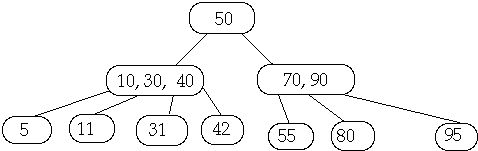Insertion in (2,4) Tree, Insert 6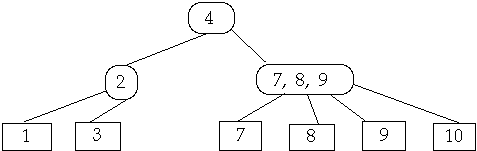Find Proper Leaf Location and Add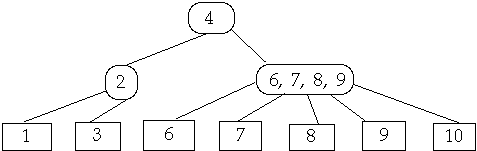If needed Split Node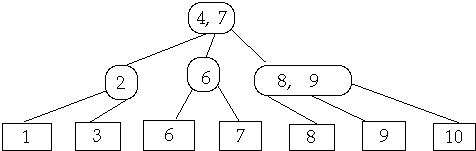Delete 6Find and Delete leaf, Shrink ParentIf needed either fuse parent orShare nodes from Sibling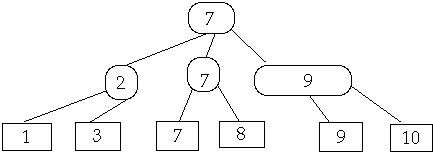Let T be an ( a, b )-tree with n leaves and height h. Then:

a)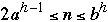b) lg (n)/lg (b) <= h <= 1 + lg( n/2 ) / log ( a )

Theorem Let b >= 2a and a >= 2. Perform any sequence of i insertions and d deletions( n = i + d ) into an initially empty ( a, b)-tree. Let
SP = total number of node splittings
F = total number of node fusings
SH = total number of node sharings

then:
SH <= d <= n
(2c - 1) SP + cF <= n + c + c (i - d - 2) / ( a + c - 1 )
where:Note c >= 1 so we have SP + F <= n/c + 1 + (n - 2 ) / a

Corollary SP + F + SH = O( n ).

This is not true when b = 2a - 1. That is for B-trees!
Values of a and b?

Assume b = 2a

Assume it costs C1 + C2m time units to move m contiguous elements from secondary to main memory

C1 = latency time, C2 = time to move one storage location

Assume it costs K1 + K2n to determine the subtree of interest in a node containing n keys

Total search time in (a , b ) -tree will be bound by
( K1 + K2a + C1 + C2a ) lg( n ) / lg( a )

This is minimal when
a* ln( a - 1 ) = ( K1 + C1 ) / ( K2 + C2 )

Tree In Main Memory

K1 ~ K2 ~ C1 and C2 = 0 so a = 2 or 3

Tree In Secondary Memory

K1 ~ K2 ~ C2 and C1 ~ 1000K1 ( in 1983 )

This gives a ~ 100
The Action is Near the Leaves

Let leaves be level 0
Parents of leaves be level 1, ...

Theorem Let b >= 2a and a >= 2. Perform any sequence of i insertions and d deletions( n = i + d ) into an initially empty ( a, b)-tree. Let
SPh = total number of node splittings at height h
Fh = total number of node fusings at height h
SHh = total number of node sharings at height h

then:
SPh + SHh + Fh <= 2( c + 2 ) n / (c + 1 ) h
where: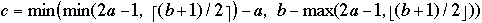( a, b )-Trees and Sorting

Let x, x, ..., x[n] be a sequence to be sorted

f[i] = | { x[j] : j > k and x[j] < x[k] } |

Let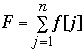F is the number of inversions of x, x, ..., x[n]

Sort x, x, ..., x[n] by inserting into a ( a, b )-Tree

But start insertions at the leaves.

theorem A sequence of n elements with F inversions can be sorted in:
O( n + n lg( F / n ) )